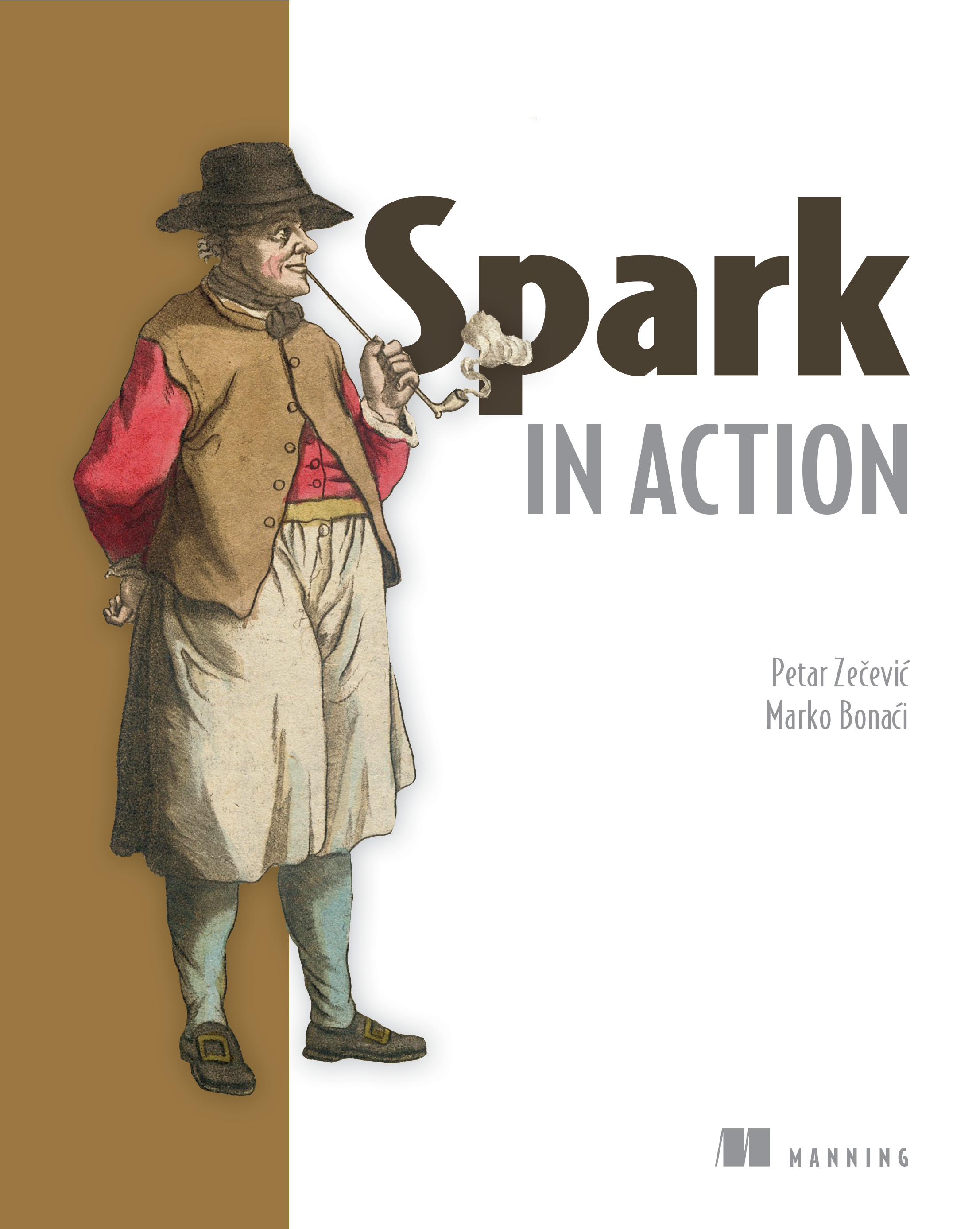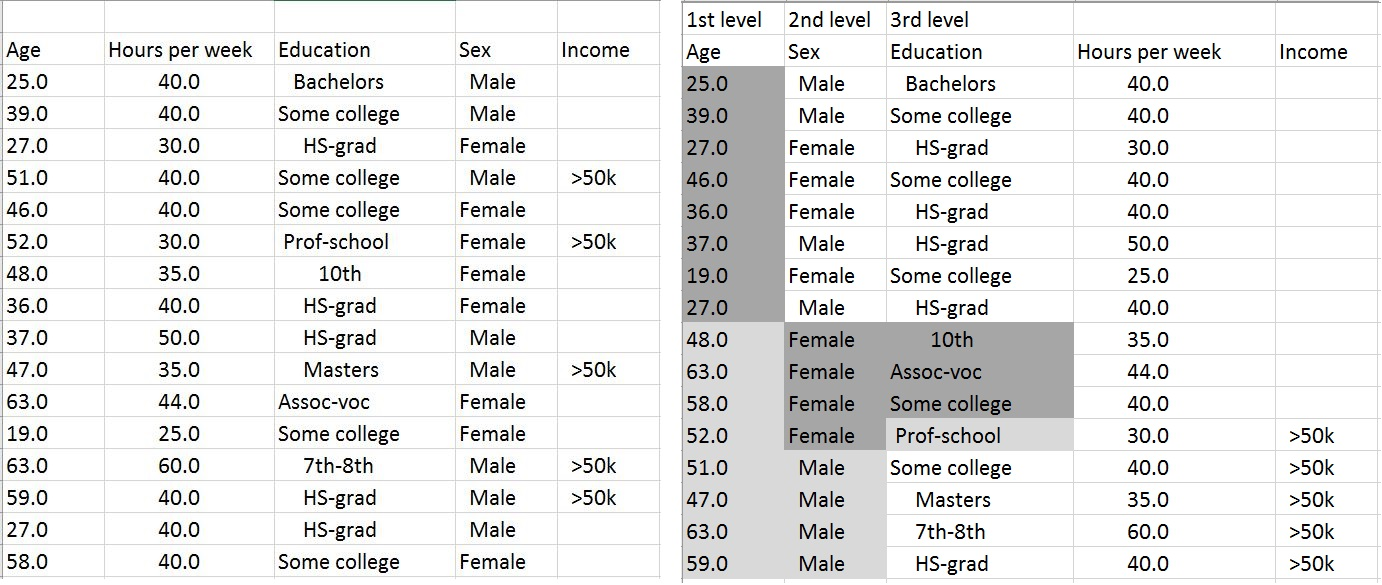Errata: October 24, 2018

Thank you for purchasing Spark in Action. Please post any errors, other than those listed below, in the book's Author Online Forum. We'll update this list as necessary. Thank you!

## Page 15

The following line should be added after the command:

"If you are referencing the json file by an absolute path, please make sure that the path doesn't contain any spaces."

## Page 16

At the beginning of the page, before the word "Finally," add the following:

The previous command created a file called Vagrantfile in the current folder. To avoid potential problems with running the VM, check if the following line is present in the file:

config.vm.network "private_network", ip: "192.168.10.2"
config.vm.network "public_network"

If not, add it to the file.

## Page 19

"Scala in Action (Manning, 2013)," should read "Scala in Action, Third Edition (Manning, 2016)."

The "vagrant ssh" command should read "vagrant ssh -- -l spark."

## Page 22

"See Appendix B for details" should read "See Appendix A for details."

## Page 30

"(not spark-shell)" should read "inside the VM (not spark-shell)."

## Page 55

Code line

``import sqlContext.implicits._``
``import spark.implicits._``
.

## Page 63

Outline note title should be changed from "Pasting blocks of code into the Spark Scala shell" to "Submitting Spark Python applications."

## Page 69

In the last line on this page, replace `map` with `values`.

...`map` and `sum` are Scala's standard methods and aren't part of Spark's API.

should be:

`...values` and `sum` are Scala's standard methods and aren't part of Spark's API.

## Page 106

The reference at the top of the second paragraph is 5.2, but it should be 5.3. The next refence to section 5.3 should be section 5.4.

## Page 109

``val spark = SparkSession.builder().getOrElse()``

``val spark = SparkSession.builder().getOrCreate()``

## Page 125

"SQLfunctions and UTFs" should read "SQLfunctions and UDFs"

## Page 125

The title of section 5.1.6 should be "Grouping data."

## Page 142, figure 5.5

The label "Analyzed local plan" should read "Analyzed logical plan."

## Page 163

The snippet at the top has an incorrect reference. In the third line,

``reduceByKey``
should be

``reduceByKeyAndWindow``
.

## Page 166

The second snippet has an incorrect reference. In the seventh line, `metric` should be `metrics`.

## Pages 169 and 170

The referencesto chapter 4 in the second half of page 169 and the top of page 170 should be chapter 2.

## Page 169

Transpose the two code annotations at the bottom of the page.

## Page 196

In the third paragraph, the text following the code callout "where n, in this example, is equal to 12" is incorrect. The correct value is 13.

## Page 198

In the first partial derivate formula on the left-hand side, wi should be wj. In the third paragraph, the reference to the black line in figure 7.6 should reference the white line.

## Page 205

...model would be move focused

...model would be more focused

## Page 208

``housingHP.first().features.count()``

``housingHP.first().features.>size``

## Page 208

``````val scalerHP = new StandardScaler(true, true)
scalerHP.fit(housingHPTrain.map(x => x.features)) ``````

``val scalerHP = new StandardScaler(true, true).fit(housingHPTrain.map(x => x.features))``

## Page 212

"||x||" symbol in the formula should be changed to "||w||"

## Page 214

In the mini-batch weight updating formula, the w on the right-hand side of the equation should be wj.

## Page 219

In the fifth paragraph, the clause ...two algorithms that can be used for both classification and clustering... should read ...two algorithms that can be used for both classification and regression....

## Page 223, figure 8.2

In the graph to the right, w1 = 2, the 2 is incorrect. It should be -2.

## Page 225

The line

``map(x => x.split(", ")).``

should be

``map(x => x.split(", ").map(_.trim)).``

## Page 230

``lrmodel.weights``
should be changed to

``lrmodel.coefficients``

## Page 231

In the first sentence, "training dataset" should be validation dataset."

## Page 239

In the fifth paragraph, the phrase ...we took the housing dataset used in the previous chapter... should be ...we took the adult dataset used in this chapter.

The same paragraph references `education`; those references should be to `age`.

## Page 240, figure 8.8

The label "<48" should be "<=48."

The last sentence in the continued paragraph (from page 239) should read "The resulting left branch only contains the negative examples (income is less than \$50,000), so it's declared to be a leaf node (the final node in the tree)."

The full corrected paragraph follows: We next used the dataset to train a decision-tree model. The way the algorithm used the original dataset is shown on at right in the figure. In the first step (in the root node of the resulting tree), the algorithm determined that the age feature should be selected first (that is why the age column is shown first in the table on the right). It divided the possible categories of the age feature according to target class values (income) into left categories, corresponding to the left branch in figure 8.8 and depicted with a black background in figure 8.7; and right categories, corresponding to the right branch in figure 8.8 and depicted with a gray background in figure 8.7. The resulting left branch only contains the negative examples (income is less than \$50,000), so it's declared to be a leaf node (the final node in the tree).

Replace figure 8.8 with the following:Also, replace figure 8.7's caption with the following: "The example dataset on the left serves for training of a decision-tree model. The model's algorithm first divides the dataset by age. The resulting right branch (gray background) is divided by sex, and the resulting left branch (black background) is divided by education. The built model (tree) has a depth of three and has seven nodes. Each group of colored cells in a column corresponds to a node, plus the root node not visible in the figure."

In the first paragraph, make the following changes:
Line 1: replace `age` with `sex`.
Line 3: replace left `education` with right `age`.
Line 4: replace right `education` with left `age`.
Line 6: remove the third sentence.
Line 9: replace left with right.
Line 11: replace `false` with `true`.
Line 11: replace right with left.
Line 12: replace `sex` with `educataion`.

The full corrected paragraph follows:
In the next step, the algorithm uses the sex feature. It repeats the same process and divides only those column values in the right age branch (because the values in the left age branch became a leaf node) into two sets. This time the right branch becomes a leaf node (predicting the classification value true). For the left branch, the education feature is chosen, and the two final leaf nodes result. As you can see, the algorithm never got to use the hours per week feature, so that feature will have no influence on predictions. The corresponding decision tree is shown in figure 8.8.

Replace figure 8.8 with the following:## Page 241

Please ignore the referencees to the gray arrows in the first sentence. It should read, "Nodes with a white background in figure 8.8 correspond to the columns used to split the dataset, and the nodes with dark gray and light gray backgrounds are leaf nodes containing final prediction values."

The entropy formula has an incorrect reference: j=i should be j=1.

The last sentence in the next to the last paragraph "For the example, the dataset in figure 8.8 is equal to 0.4296875" should read "For the example dataset in figure 8.8, Gini impurity is equal to 0.4296875."

## Page 242

The second formula should be IG(D, Fage) = 0.896038 - 8/16 (- 5/8 log2 5/8 - 3/8 log2 3/8) - 8/16 0 =0.4188205.

The paragraph just before this formula contains the phrase "... when splitting the dataset according to the feature education." This should read "...when splitting the dataset according to the feature age."

The last paragraph should read: "If any other split of age values were chosen, information gain would be lower. This is obvious by examining the age and income columns in figure 8.7. If the chosen condition for dividing age column values was "<=47" instead of "<=46", one row having class of ">\$50k" would be moved to the left branch, which would no longer contain a single class. That would cause left branch's impurity to be greater than zero and thus reduce information gain. The algorithm uses information gain in this way to decide how to split the dataset at each node of the decision tree."

## Page 343

The parameter is spelled wrong in the line, "The directory for storing log files is determined by the parameter `yarn.nodeamanager.log-dirs` (set in yarn-site.xml), which defaults to /logs/userlogs." It contains an erroneous a; it should be `yarn.nodemanager`.

## Page 419

Matrix addition result should be changed from:
a11 + b12 a12+b12
a21 + b22 a21+b22
to:
a11 + b11 a12+b12
a21 + b21 a22+b22

## Index

Beyond DataFrames: introducing DataSets is listed in Section 5.7 incorrectly. Remove this entry. The correct section is 5.2.